# Poetry in Motion

Phil Ramsden gave an excellent talk at the 2013 MathsJam conference, about a particularly mathematical form of poetry. We asked him to write an article explaining it in more detail.

Generals gathered in their masses,
Just like witches at black masses.

(Butler et al., “War Pigs”, Paranoid, 1970)

Brummie hard-rockers Black Sabbath have sometimes been derided for the way writer Geezer Butler rhymes “masses” with “masses”. But this is a little unfair. After all, Edward Lear used to do the same thing in his original limericks. For example:

There was an Old Man with a beard,
Who said, “It is just as I feared!-
Two Owls and a Hen,
Four Larks and a Wren,
Have all built their nests in my beard!”

(“There was an Old Man with a beard”, from Lear, E., A Book Of Nonsense, 1846.)

And actually, the practice goes back a lot longer than that. The sestina is a poetic form that dates from the 12th century, and was later perfected by Dante. It works entirely on “whole-word” rhymes.

A sestina has thirty-nine lines. The main (and mathematically interesting) part of the poem consists of six stanzas of six lines each; there’s then a three-line envoi. I wrote a sestina for the 2013 MathsJam conference; the full poem, in all its dubious glory, is available on request, but here are the first two stanzas:

One Saturday, with gladdened heart I gaily rise
And greet the Autumn morn at this unwonted hour
With strong black coffee’s aid, I slowly come to life
I shower, shave and dress, bolt down some bread and jam
Pick up my case; take laptop-holder on my back;
And then anon to Turnpike Lane direct my steps.

At Euston station, as I ride the rolling steps
That seem to mumble, groan and mutter as they rise
I muse: it is another year, and we are back!
As time goes by, it seems it quickens by the hour
The world’s events now seem to cluster, crowd and jam
Accelerating as they touch my fleeting life.

As you can see, the rhyming happens not within stanzas but between them. The six “rhyme words” that appear at the ends of the lines of the first stanza also appear in all the others. The words are the same, but the order is different: they’ve been permuted from stanza to stanza. The same permutation is used each time, so all in all, the rhyme scheme looks like this: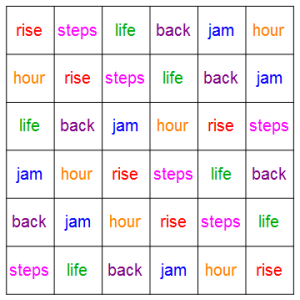Or, more abstractly: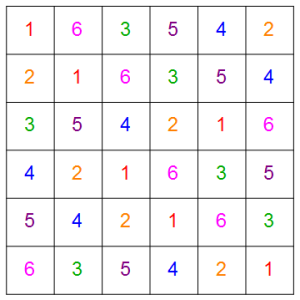This permutation is most easily studied by focusing on its inverse, which is given by

$\delta_6(u) = \left\{\begin{array}{rc}2\,u,&\quad 1\le u \le 3,\\13-2\,u,&\quad \text{otherwise.}\end{array}\right.$

This inverse permutation (and therefore the original permutation) has order $6$: that’s to say, ${\delta_6}^6(u) \equiv u$ (and ${\delta_6}^n(u)$ is not equal to $u$ for any value of $n$ smaller than $6$). Moreover, it’s a complete cycle: the sequence

$1, \delta_6(1), {\delta_6}^2(1), \dots, {\delta_6}^5(1)$

goes through all the numbers from $1$ to $6$. It’s this feature that makes it work as a rhyme-scheme.

Sestinas aren’t unique. There’s such a thing, for example, as a quintina, the main part of which consists of five stanzas of five lines each. The quintina’s rhyme-scheme looks like this: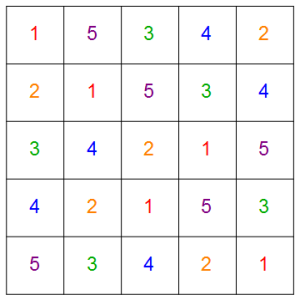This corresponds to the inverse permutation:

$\delta_5(u) = \left\{\begin{array}{rc}2\,u,&\quad 1\le u \le 2,\\11-2\,u,&\quad \text{otherwise.}\end{array}\right.$

In general, we can talk about the $n$-tina, and its inverse permutation:

$\delta_n(u) = \left\{\begin{array}{rc}2\,u,&\quad 1\le u \le n/2,\\2\,n+1-2\,u,&\quad \text{otherwise.}\end{array}\right.$

But not all $n$-tinas make good rhyme-schemes. That’s because the permutation isn’t always a complete cycle. For example, in the case of the ottina (which, if it existed, would consist in the main of eight stanzas of eight lines), the permutation looks like this: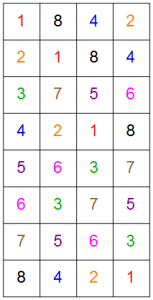As you can see, it consists of two disjoint cycles of order $4$ (namely $(1842)$ and $(3756)$). This means that the permutation itself has order $4$: our poem would have the same order of rhyme-words in the fifth stanza as in the first (for example).

The settina ($n=7$) is even worse. Here’s its permutation: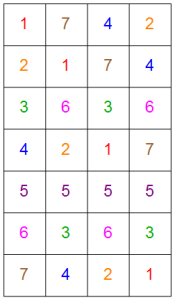This resolves into the cycles $(1742)$, $(36)$ and (worst of all), $(5)$: the fifth line’s rhyme word simply stays the same from stanza to stanza! Overall, the permutation has order $4$, which doesn’t even divide the number of stanzas, $7$. This would be spectacularly unsuitable as a rhyme scheme.

The literature on sestinas somtimes calls values of $n$ for which the $n$-tina permutation is a complete cycle admissible numbers; this struck me as a little uninspired given the subject matter. Elsewhere, they’re called Queneau numbers, after the poet and mathematician (yes, really) Raymond Queneau, who seems to have been the first to pose mathematical problems about sestinas. In my MathsJam talk, I opted to break with this terminology, and instead call such numbers sheerly poetic (which allows us to say that numbers like this exhibit the property of sheer poetry).

It’s known that $n$ is sheerly poetic if and only if the corresponding permutation has order $n$. Now, this isn’t true of permutations in general: for example, the permutation $(123)(45)(6)$ has order $6$, but it isn’t a complete cycle. However, within the restricted class we’re interested in, all permutations of $n$ elements that are of order $n$ really are complete cycles.

Here are the first few sheerly poetic numbers:

$n=1,2,3,5,6,9,11,14,18,23,26,29,30,33,35,39,\dots$

They might not look all that familiar. That changes, though, if instead we note that

$2\,n+1=3,5,7,11,13,19,23,29,37,47,53,59,61,67,71,79,\dots,$

and indeed it’s known that if $n$ is sheerly poetic then $2\,n+1$ must be prime.

This actually isn’t that hard to prove; contact me for details! The proof, like that of nearly all theorems to do with $n$-tinas, makes use of the fact that modulo $2\,n+1$, the inverse permutation $\delta_n$ always corresponds either to multiplication by $2$ or to multiplication by $-2$.

But you’ll notice that some primes are missing from this list. For example, $17$ is certainly prime, but as we’ve already noted, $8$, equally certainly, won’t quite do as a value of $n$ to build a rhyme-scheme on (though it wouldn’t be as disastrous as $7$). So the converse of our theorem is false: if $n$ is sheerly poetic, then $2\,n+1$ is prime, but not necessarily the other way round.

So let’s call $n$ merely poetic if ${\delta_n}^n$ is the identity: that is, if the order of the permutation (or, equivalently, its inverse) divides $n$. If $n$ is merely poetic but not sheerly poetic, then you could write an authentic $n$-tina, as long as you didn’t mind the rhyme-scheme repeating itself a bit; at least, when you came to the end of your $n$ stanzas of $n$ lines, you wouldn’t be in mid-cycle.

Now, according to this definition, $8$ is merely poetic (permutation order $4$, which divides $8$), but $7$ isn’t (permutation order $4$, which doesn’t divide $7$). It turns out (and this is also known) that if $2\,n+1$ is prime, then $n$ is merely poetic (and may be sheerly poetic). Once again, the proof uses the fact that the inverse permutation $\delta_n$ corresponds, modulo $2\,n+1$, to multiplication by $\pm 2$.

Which would be quite tidy, except that the converse of this theorem is false as well. That’s to say, there are merely poetic numbers $n$ for which $2\,n+1$ simply isn’t prime. The first few of those are

$n=170, 280, 552, 864, 952, \dots,$

corresponding to

$2\,n+1=341, 561, 1105, 1729, 1905, \dots.$

Those values of $2\,n+1$ may just possibly be familiar to some; they have quite a special property. They’re all pseudoprimes to base $2$: that is, they’re all non-primes with the property that

$2^{p-1}\equiv 1 \pmod p.$

Now, all primes have this property: that’s what Fermat’s Little Theorem tells us (and an extension of this fact forms the basis of RSA public-key encryption, on which Internet commerce is based). But some exceptional composite numbers also have it, including those above. That prompts the following conjecture:

If $n$ is merely poetic (or sheerly poetic) then $2\,n+1$ is either a prime or a pseudoprime to base $2$.

I think I’ve proved that, and if you want to check whether you agree that I have (and I’d love you to), please contact me. But alas, the dance continues; yet again, the converse is false. There are pseudoprimes to base $2$ for which the corresponding value of $n$ isn’t merely poetic at all. One example is $645$: although it’s certainly true that $2^{644} \equiv 1 \pmod{645}$, the corresponding permutation, $\delta_{322}$, doesn’t have an order that divides $322$. (Its order is in fact $28$, which goes $11.5$ times into $322$.)

However, you’ll notice it does have an order that divides $2\times322=644$. That prompts another conjecture. Let’s call $n$ nearly poetic if ${\delta_n}^{2\,n}$ is the identity (so while you might not be able to finish your $n$-tina at the end of a cycle, you’d at least know you can finish it exactly halfway through one). Then

If $2\,n+1$ is either a prime or a pseudoprime to base $2$, then $n$ is (at least) nearly poetic.

Again, this is something I think I’ve proved. And this time, I’m pretty convinced that the converse is true: that if $n$ is at least nearly poetic, then $2\,n+1$ is either a prime or a pseudoprime to base $2$. However, I haven’t yet been able to prove the converse; if you can, I’d love to hear from you.

### Bibliography

If you want to read further on sestinas and Queneau numbers, here’s a brief bibliography. Some of the key articles are in French, and don’t seem to have been translated, but the English paper by Saclolo is an excellent overview. If you do feel like diving into one of the French ones, I’d start with the Bringer paper.

Audin, M., ‘Mathématiques et littérature‘, Mathématiques et sciences humaines, 178 (2007), pp 63-86

Bringer, M., ‘Sur un problème de R. Queneau‘, Mathématiques et sciences humaines, 27 (1969), pp 13-20

Dumas, J.-G., ‘Caractérisation des quenines et leur représentation spirale‘, Mathématiques et sciences humaines, 184 (2008), pp 9-23

Queneau, R., ‘Note complémentaire sur la sextine’, Subsidia Pataphysica 1 (1963), 79-80

Roubaud, J., ‘Un problème combinatoire posé par la poésie lyrique des troubadours‘, Mathématiques et sciences humaines, 27 (1969), pp 5-12

Saclolo, M. P., 2011, ‘How a Medieval Troubadour Became a Mathematical Figure‘, Notices of the American Mathematical Society, 58(5) (2011), pp 683-687

•